# Chapter 3 Stoichiometry Calculations with Chemical Formulas and

• Slides: 64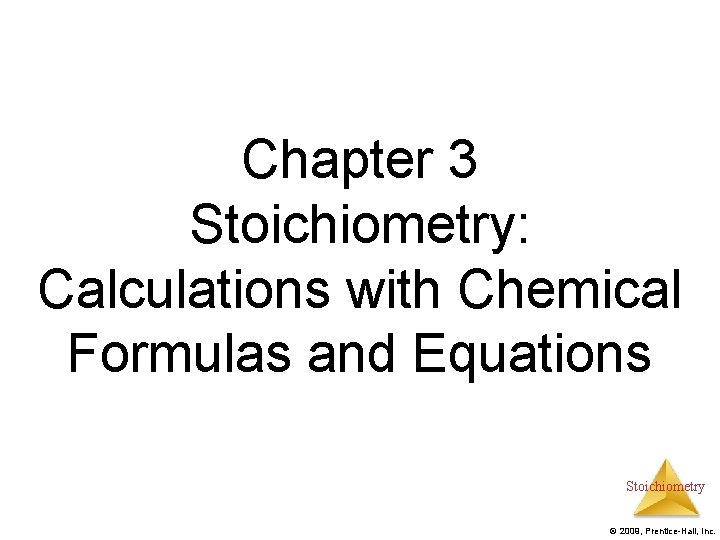Chapter 3 Stoichiometry: Calculations with Chemical Formulas and Equations Stoichiometry © 2009, Prentice-Hall, Inc.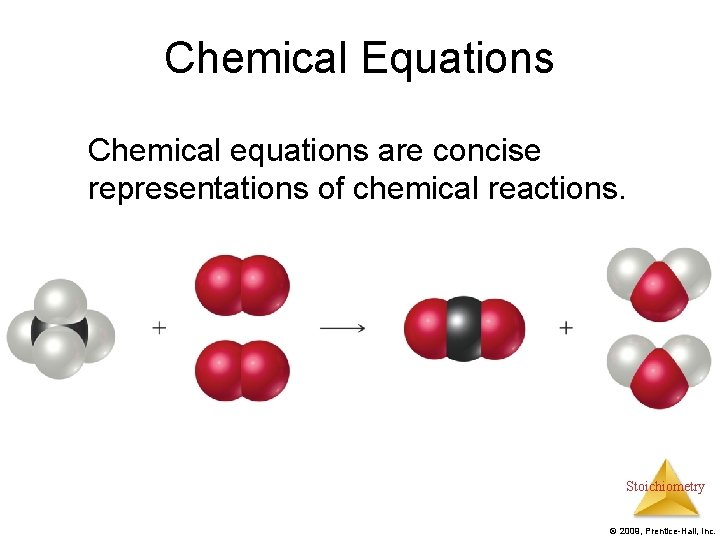Chemical Equations Chemical equations are concise representations of chemical reactions. Stoichiometry © 2009, Prentice-Hall, Inc.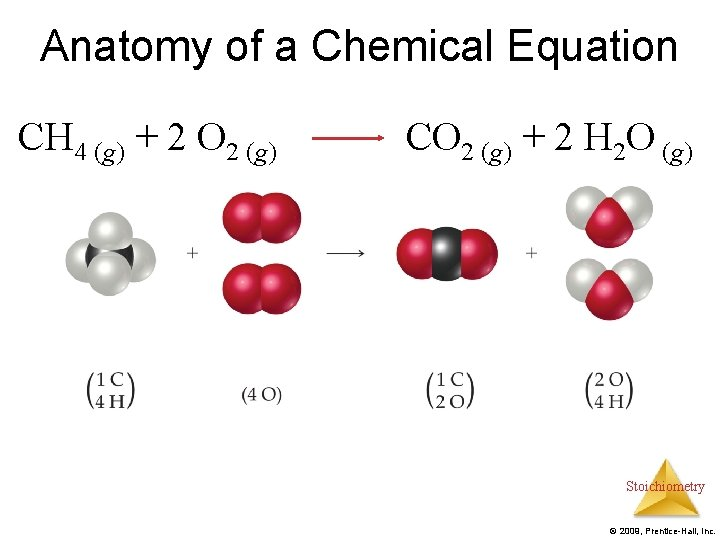Anatomy of a Chemical Equation CH 4 (g) + 2 O 2 (g) CO 2 (g) + 2 H 2 O (g) Stoichiometry © 2009, Prentice-Hall, Inc.Anatomy of a Chemical Equation CH 4 (g) + 2 O 2 (g) CO 2 (g) + 2 H 2 O (g) Reactants appear on the left side of the equation. Stoichiometry © 2009, Prentice-Hall, Inc.Anatomy of a Chemical Equation CH 4 (g) + 2 O 2 (g) Products appear on the right side of the equation. CO 2 (g) + 2 H 2 O (g) Stoichiometry © 2009, Prentice-Hall, Inc.Anatomy of a Chemical Equation CH 4 (g) + 2 O 2 (g) CO 2 (g) + 2 H 2 O (g) The states of the reactants and products are written in parentheses to the right of each compound. Stoichiometry © 2009, Prentice-Hall, Inc.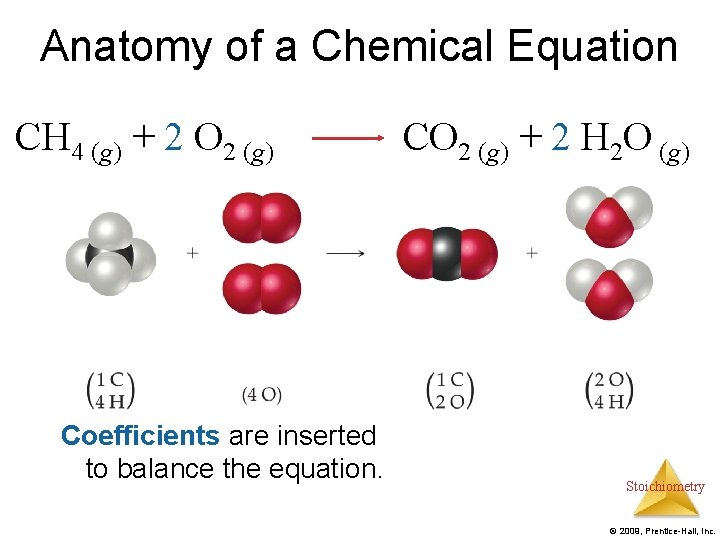Anatomy of a Chemical Equation CH 4 (g) + 2 O 2 (g) Coefficients are inserted to balance the equation. CO 2 (g) + 2 H 2 O (g) Stoichiometry © 2009, Prentice-Hall, Inc.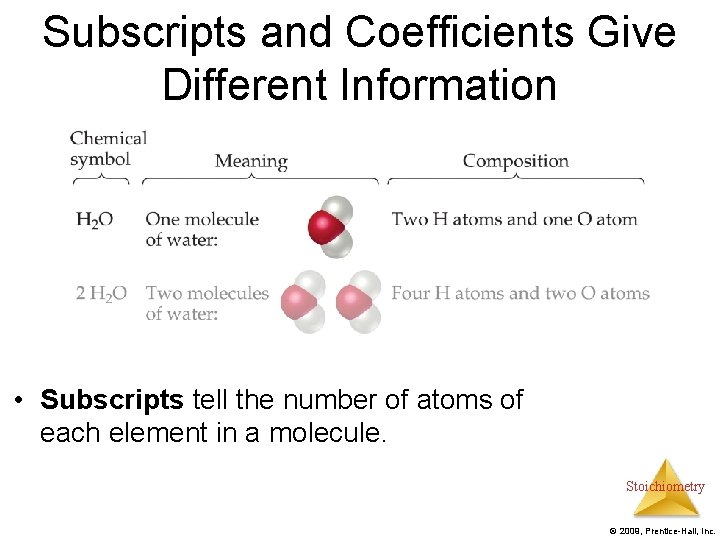Subscripts and Coefficients Give Different Information • Subscripts tell the number of atoms of each element in a molecule. Stoichiometry © 2009, Prentice-Hall, Inc.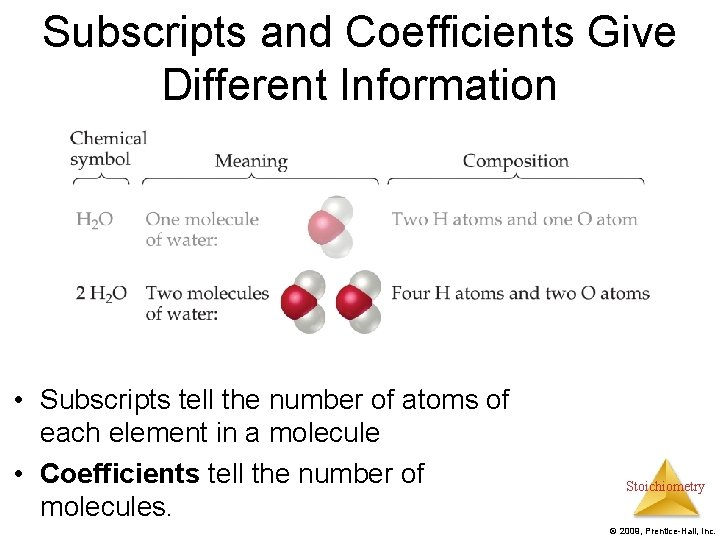Subscripts and Coefficients Give Different Information • Subscripts tell the number of atoms of each element in a molecule • Coefficients tell the number of molecules. Stoichiometry © 2009, Prentice-Hall, Inc.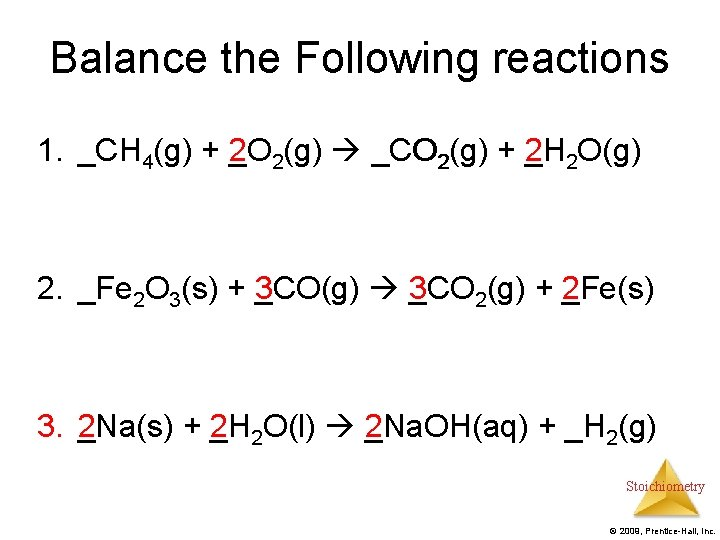Balance the Following reactions 1. CH _CH 4(g) + 2 O (g) + _O 2(g) CO (g) _CO 2(g) + 2 H (g) + _H 2 O(g) 2. _Fe 2 O 3(s) + 3 CO(g) 3 CO (s) + _CO(g) _CO 2(g) + 2 Fe(s) (g) + _Fe(s) 3. 2 Na(s) + 2 H _Na(s) + _H 2 O(l) _Na. OH(aq) + _H O(l) 2 Na. OH(aq) + _H 2(g) Stoichiometry © 2009, Prentice-Hall, Inc.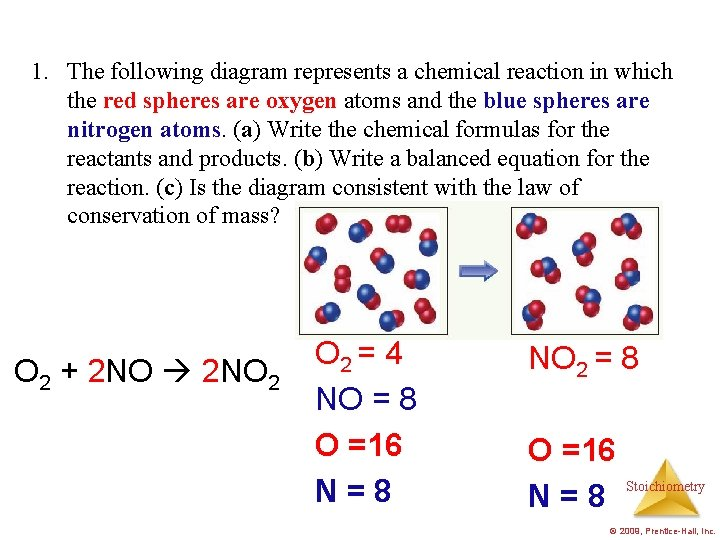1. The following diagram represents a chemical reaction in which the red spheres are oxygen atoms and the blue spheres are nitrogen atoms. (a) Write the chemical formulas for the reactants and products. (b) Write a balanced equation for the reaction. (c) Is the diagram consistent with the law of conservation of mass? O 2 + 2 NO 2 O 2 = 4 NO = 8 O =16 N=8 NO 2 = 8 O =16 N=8 Stoichiometry © 2009, Prentice-Hall, Inc.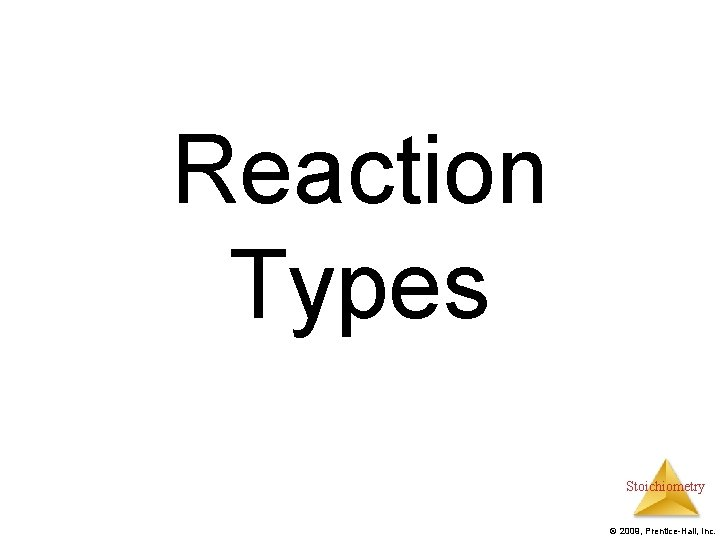Reaction Types Stoichiometry © 2009, Prentice-Hall, Inc.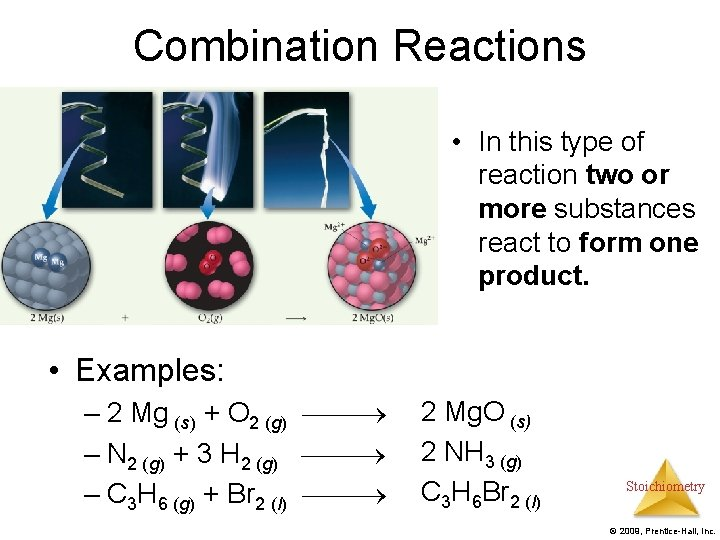Combination Reactions • In this type of reaction two or more substances react to form one product. • Examples: – 2 Mg (s) + O 2 (g) 2 Mg. O (s) – N 2 (g) + 3 H 2 (g) 2 NH 3 (g) – C 3 H 6 (g) + Br 2 (l) C 3 H 6 Br 2 (l) Stoichiometry © 2009, Prentice-Hall, Inc.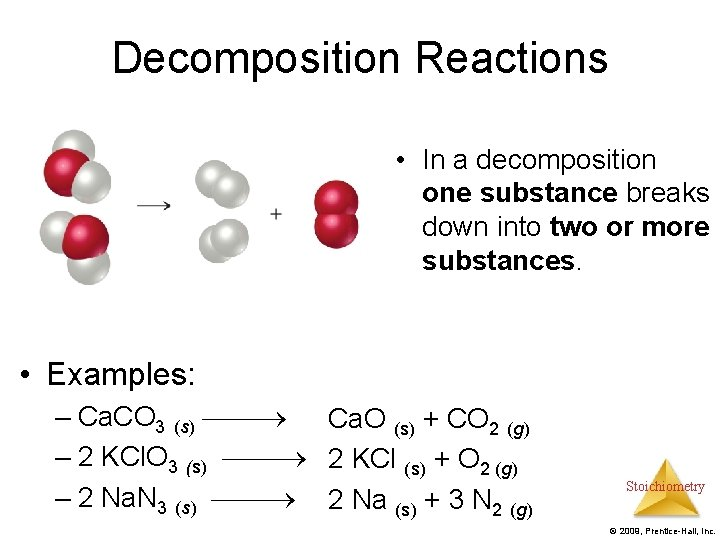Decomposition Reactions • In a decomposition one substance breaks down into two or more substances. • Examples: – Ca. CO 3 (s) Ca. O (s) + CO 2 (g) – 2 KCl. O 3 (s) 2 KCl (s) + O 2 (g) – 2 Na. N 3 (s) 2 Na (s) + 3 N 2 (g) Stoichiometry © 2009, Prentice-Hall, Inc.Combustion Reactions • These are generally rapid reactions that produce a flame. • Most often involve hydrocarbons reacting with oxygen in the air. • Examples: – CH 4 (g) + 2 O 2 (g) CO 2 (g) + 2 H 2 O (g) – C 3 H 8 (g) + 5 O 2 (g) 3 CO 2 (g) + 4 H 2 O (g) Stoichiometry © 2009, Prentice-Hall, Inc.Formula Weights Stoichiometry © 2009, Prentice-Hall, Inc.Formula Weight (FW) • A formula weight or Formula massis the sum of the atomic weights for the atoms in a chemical formula. • So, the formula weight of calcium chloride, Ca. Cl 2, would be Ca: 1(40. 1 amu) + Cl: 2(35. 5 amu) 111. 1 amu • Formula weights are generally reported for ionic compounds. Stoichiometry © 2009, Prentice-Hall, Inc.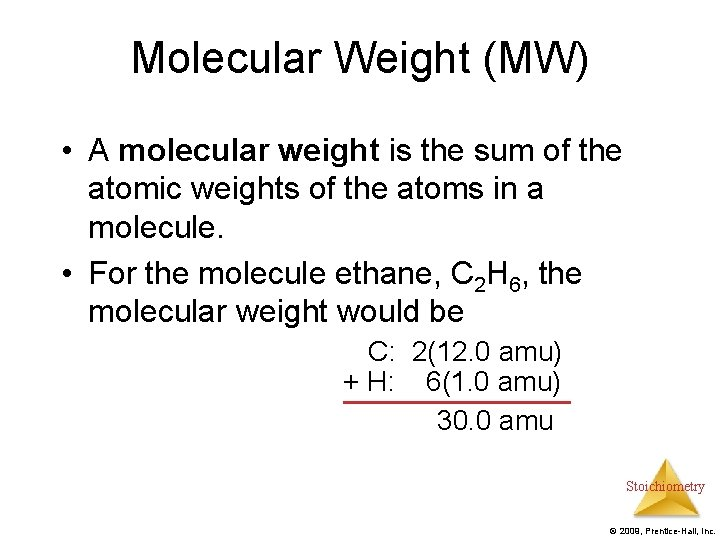Molecular Weight (MW) • A molecular weight is the sum of the atomic weights of the atoms in a molecule. • For the molecule ethane, C 2 H 6, the molecular weight would be C: 2(12. 0 amu) + H: 6(1. 0 amu) 30. 0 amu Stoichiometry © 2009, Prentice-Hall, Inc.Examples Find formula weight: a. Ca(NO 3)2 b. CH 3 OH a. 1 Ca atom = 1(40. 1 amu) = 40. 1 amu 2 N atom = 2(14. 0 amu) = 28. 0 amu 6 O atom = 6(16. 0 amu) = 96. 0 amu 164. 1 amu Stoichiometry © 2009, Prentice-Hall, Inc.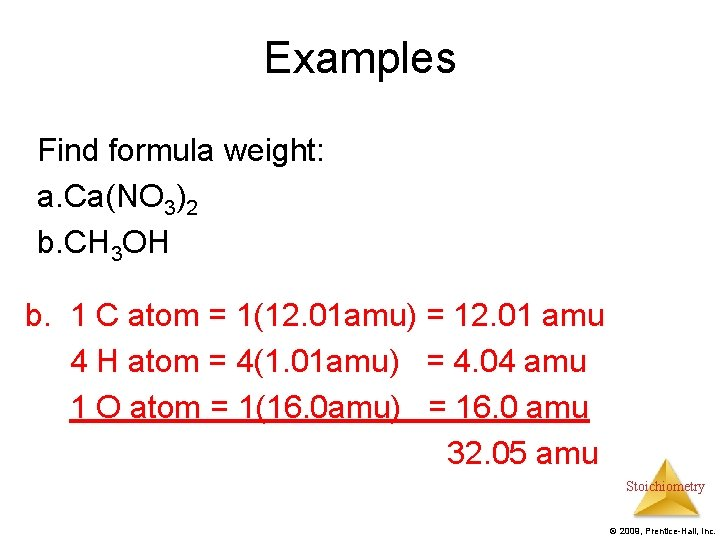Examples Find formula weight: a. Ca(NO 3)2 b. CH 3 OH b. 1 C atom = 1(12. 01 amu) = 12. 01 amu 4 H atom = 4(1. 01 amu) = 4. 04 amu 1 O atom = 1(16. 0 amu) = 16. 0 amu 32. 05 amu Stoichiometry © 2009, Prentice-Hall, Inc.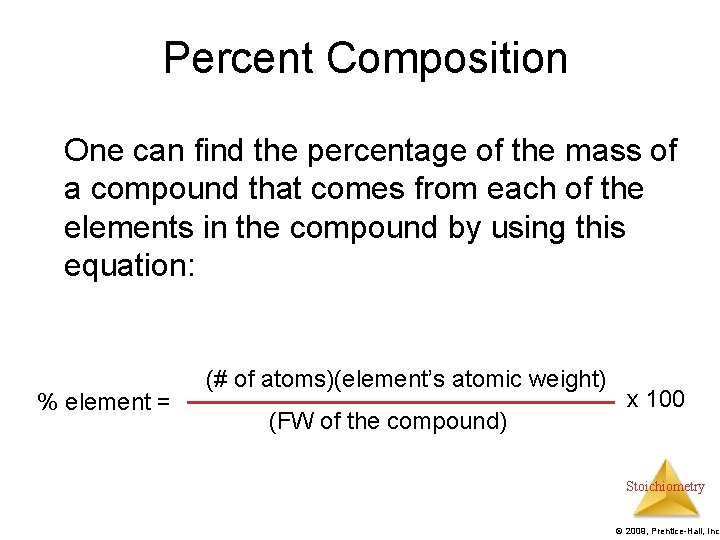Percent Composition One can find the percentage of the mass of a compound that comes from each of the elements in the compound by using this equation: % element = (# of atoms)(element’s atomic weight) (FW of the compound) x 100 Stoichiometry © 2009, Prentice-Hall, Inc.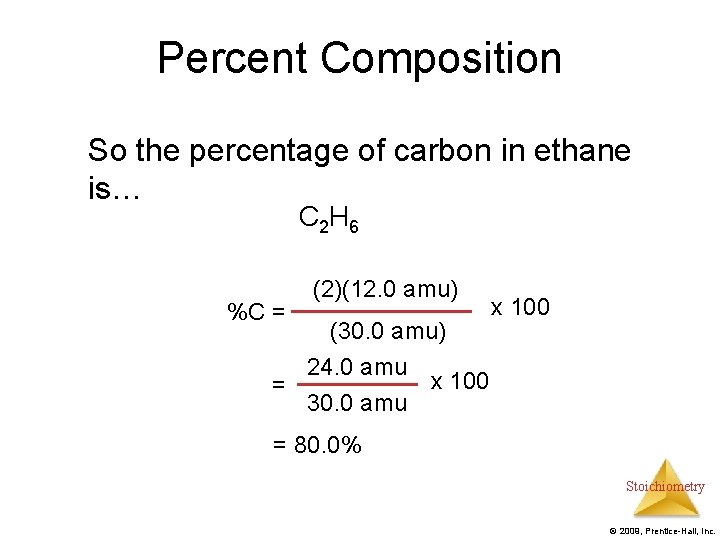Percent Composition So the percentage of carbon in ethane is… C 2 H 6 %C = (2)(12. 0 amu) (30. 0 amu) 24. 0 amu x 100 = 30. 0 amu x 100 = 80. 0% Stoichiometry © 2009, Prentice-Hall, Inc.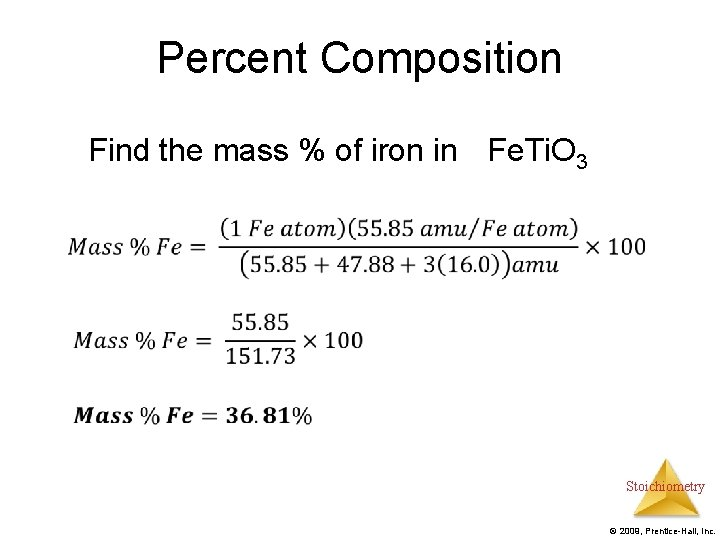Percent Composition Find the mass % of iron in Fe. Ti. O 3 Stoichiometry © 2009, Prentice-Hall, Inc.Percent Composition Find the mass % of Titanium in Fe. Ti. O 3 Stoichiometry © 2009, Prentice-Hall, Inc.Percent Composition Find the mass % of oxygen in Fe. Ti. O 3 Check: 36. 81% + 31. 56% + 31. 64% = 100. 01% Stoichiometry © 2009, Prentice-Hall, Inc.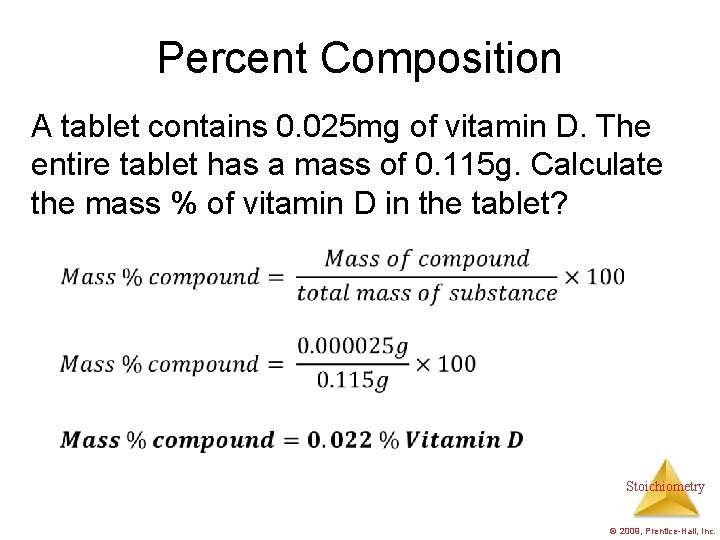Percent Composition A tablet contains 0. 025 mg of vitamin D. The entire tablet has a mass of 0. 115 g. Calculate the mass % of vitamin D in the tablet? Stoichiometry © 2009, Prentice-Hall, Inc.Percent Composition A sample of potassium permanganate (KMn. O 4) is known to contain some impurities. It is found that K+ makes up 19. 24% of the entire mass of the sample. All of the K+ comes from the KMn. O 4 compound. Find the mass % of KMn. O 4 in the sample. Stoichiometry © 2009, Prentice-Hall, Inc.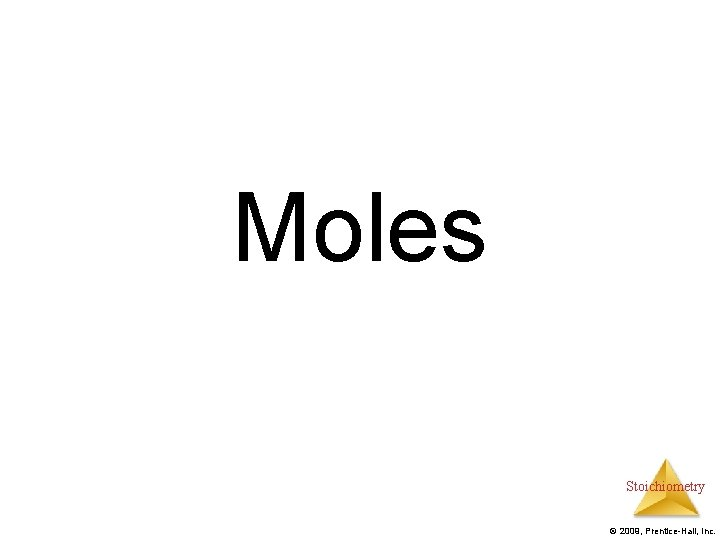Moles Stoichiometry © 2009, Prentice-Hall, Inc.Avogadro’s Number Na 6. 02 x 23 -1 10 mol Also amu/g Stoichiometry © 2009, Prentice-Hall, Inc.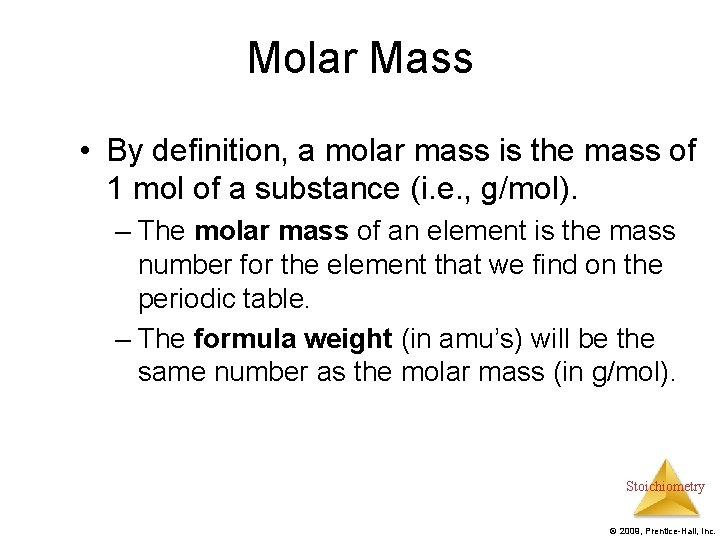Molar Mass • By definition, a molar mass is the mass of 1 mol of a substance (i. e. , g/mol). – The molar mass of an element is the mass number for the element that we find on the periodic table. – The formula weight (in amu’s) will be the same number as the molar mass (in g/mol). Stoichiometry © 2009, Prentice-Hall, Inc.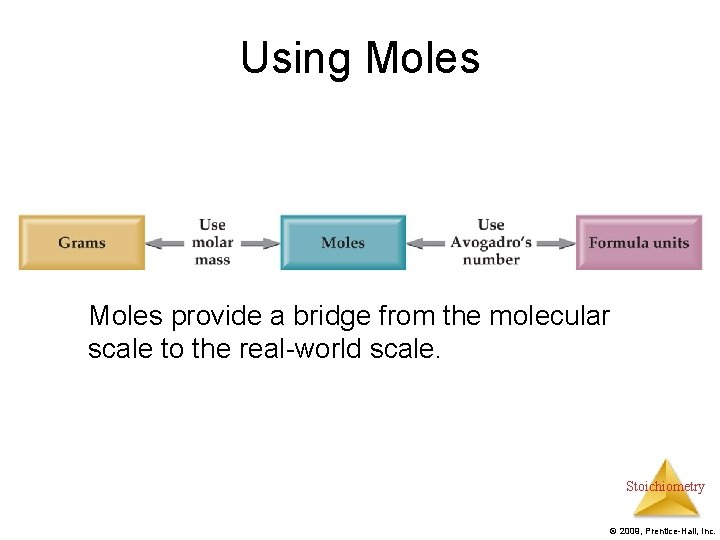Using Moles provide a bridge from the molecular scale to the real-world scale. Stoichiometry © 2009, Prentice-Hall, Inc.Converting Grams to Moles How many moles of Na. Cl are there in 48 g of Na. Cl? Stoichiometry © 2009, Prentice-Hall, Inc.Converting Moles to Grams How many grams of C 2 H 6 are there in 18. 7 moles of C 2 H 6? Stoichiometry © 2009, Prentice-Hall, Inc.Converting Grams to Molecules 1. How many water molecules are contained within a 56 g pure sample of water? 2. How many hydrogen atoms are combines with in 56 g pure sample of water? StoichiometryFinding Empirical Formulas Stoichiometry © 2009, Prentice-Hall, Inc.Calculating Empirical Formulas One can calculate the empirical formula from the percent composition. Stoichiometry © 2009, Prentice-Hall, Inc.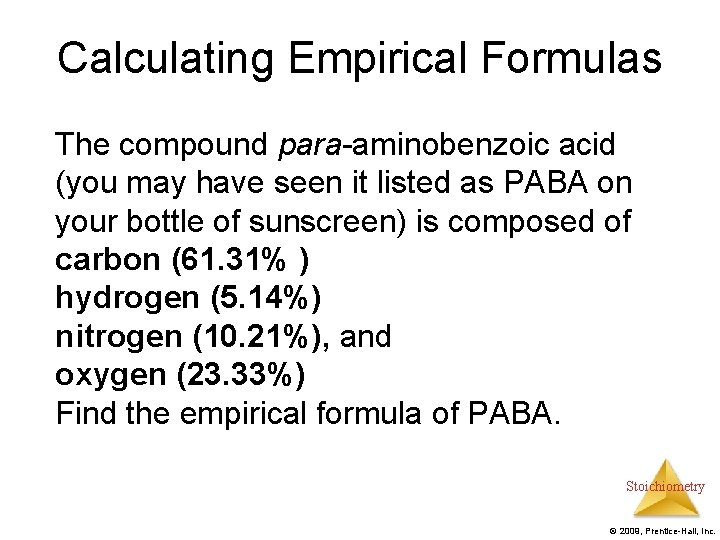Calculating Empirical Formulas The compound para-aminobenzoic acid (you may have seen it listed as PABA on your bottle of sunscreen) is composed of carbon (61. 31% ) hydrogen (5. 14%) nitrogen (10. 21%), and oxygen (23. 33%) Find the empirical formula of PABA. Stoichiometry © 2009, Prentice-Hall, Inc.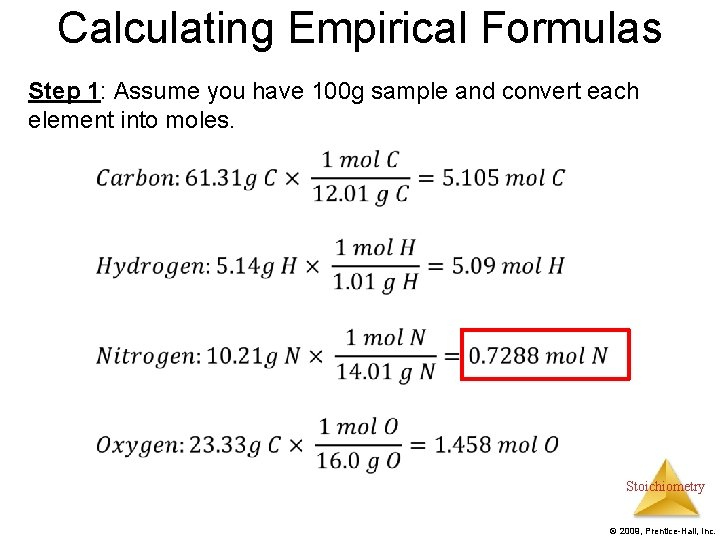Calculating Empirical Formulas Step 1: Assume you have 100 g sample and convert each element into moles. Stoichiometry © 2009, Prentice-Hall, Inc.Calculating Empirical Formulas Step 2: Calculate the mole ratio by dividing by the smallest number of moles: C: 5. 105 mol 0. 7288 mol H: 5. 09 mol 0. 7288 mol = 6. 984 7 N: 0. 7288 mol = 1. 000 O: 1. 458 mol 0. 7288 mol = 2. 001 2 = 7. 005 7 Empirical Formula: C 7 H 7 NO 2 Stoichiometry © 2009, Prentice-Hall, Inc.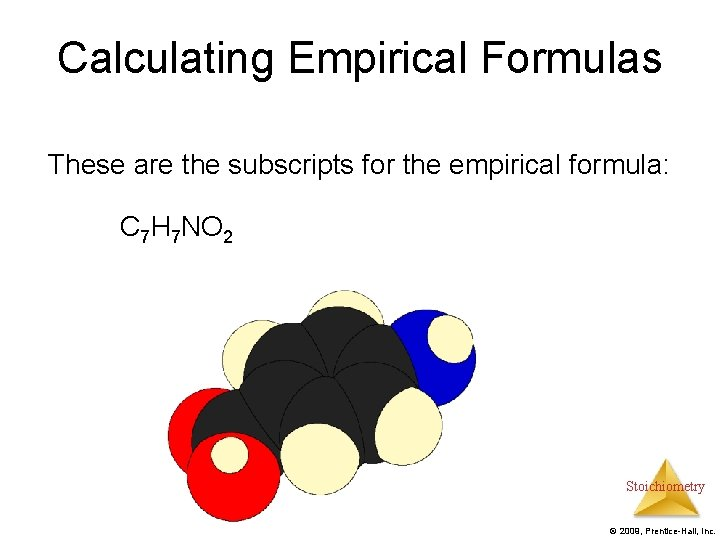Calculating Empirical Formulas These are the subscripts for the empirical formula: C 7 H 7 NO 2 Stoichiometry © 2009, Prentice-Hall, Inc.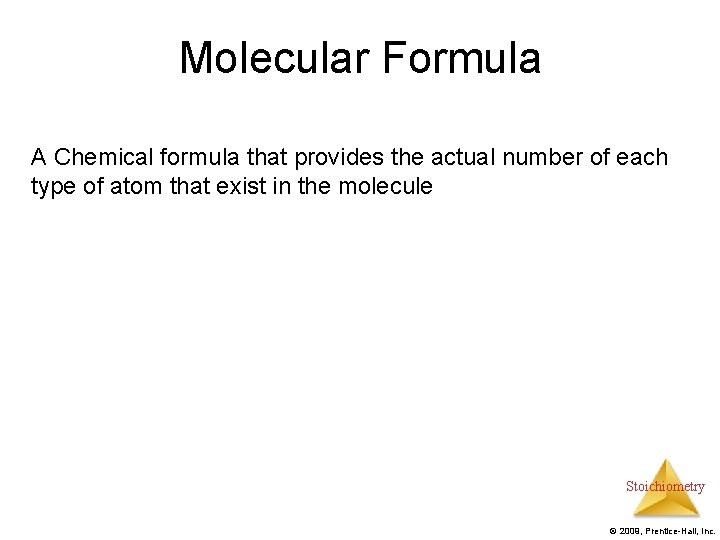Molecular Formula A Chemical formula that provides the actual number of each type of atom that exist in the molecule Stoichiometry © 2009, Prentice-Hall, Inc.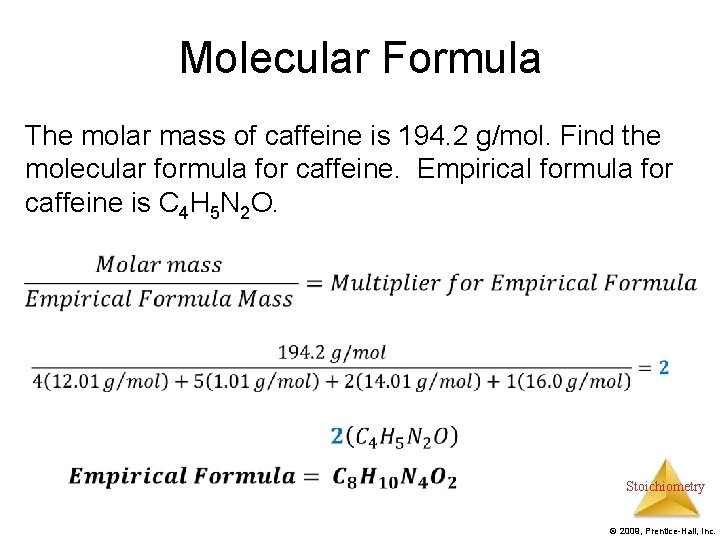Molecular Formula The molar mass of caffeine is 194. 2 g/mol. Find the molecular formula for caffeine. Empirical formula for caffeine is C 4 H 5 N 2 O. Stoichiometry © 2009, Prentice-Hall, Inc.Combustion Analysis • Compounds containing C, H and O are routinely analyzed through combustion in a chamber like this. – C is determined from the mass of CO 2 produced. – H is determined from the mass of H 2 O produced. – O is determined by difference after the C and H have been determined. • Can determine %composition and empirical formula Stoichiometry © 2009, Prentice-Hall, Inc.Combustion Analysis Example: A 2. 04 g sample containing C, H, and O underwent combustion analysis. 4. 48 g of CO 2 and 2. 4 g H 2 O were produced. Find the empirical formula. Step 1: Use stoichiometry to find the grams of Carbon and Hydrogen. Subtract the total mass from the sum of the masses of carbon and hydrogen to find the mass of oxygen Step 2: Find moles of carbon, hydrogen, and oxygen Step 3: Find the empirical formula Stoichiometry © 2009, Prentice-Hall, Inc.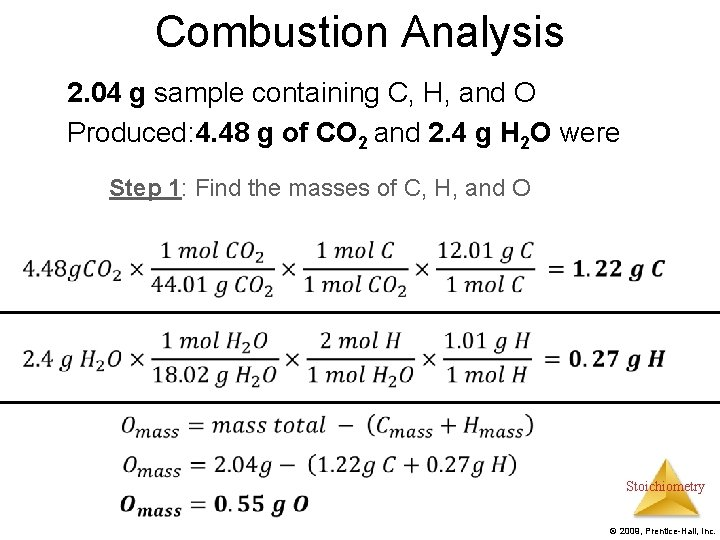Combustion Analysis 2. 04 g sample containing C, H, and O Produced: 4. 48 g of CO 2 and 2. 4 g H 2 O were Step 1: Find the masses of C, H, and O Stoichiometry © 2009, Prentice-Hall, Inc.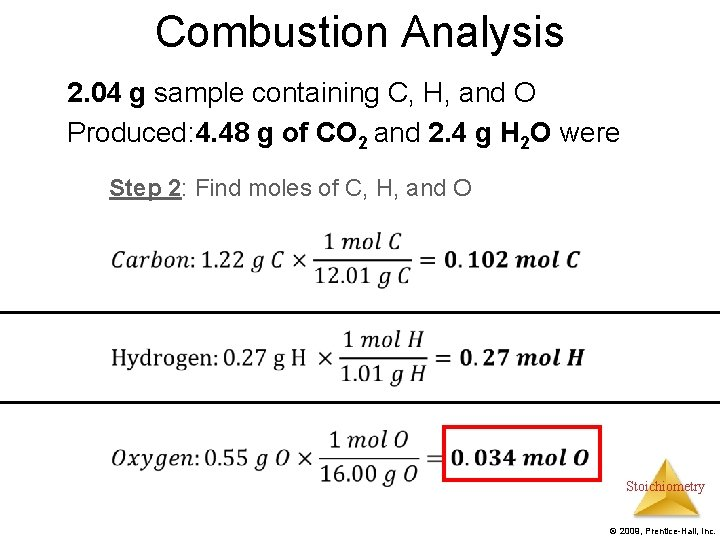Combustion Analysis 2. 04 g sample containing C, H, and O Produced: 4. 48 g of CO 2 and 2. 4 g H 2 O were Step 2: Find moles of C, H, and O Stoichiometry © 2009, Prentice-Hall, Inc.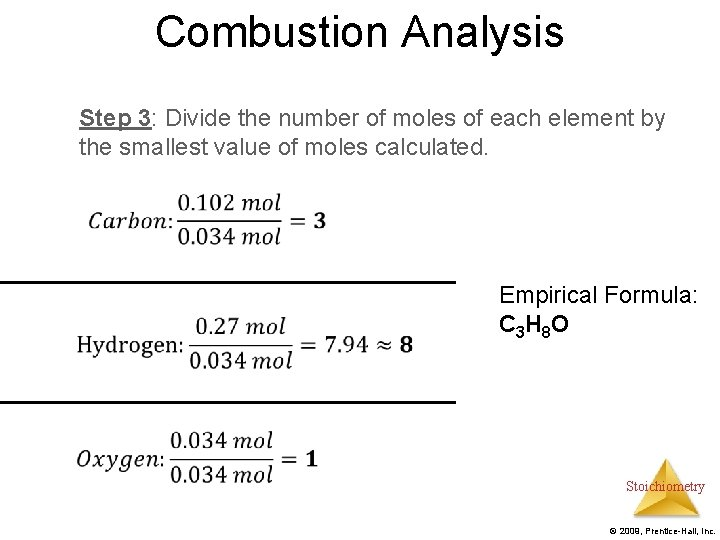Combustion Analysis Step 3: Divide the number of moles of each element by the smallest value of moles calculated. Empirical Formula: C 3 H 8 O Stoichiometry © 2009, Prentice-Hall, Inc.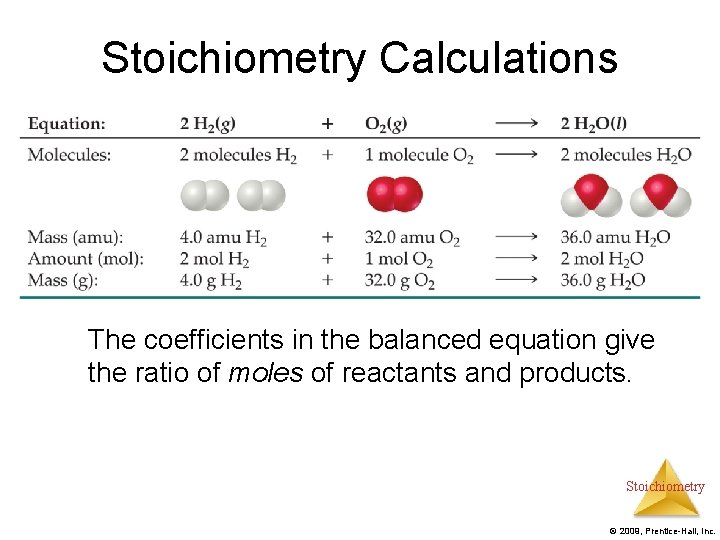Stoichiometry Calculations The coefficients in the balanced equation give the ratio of moles of reactants and products. Stoichiometry © 2009, Prentice-Hall, Inc.Stoichiometric Calculations Starting with the mass of Substance A you can use the ratio of the coefficients of A and B to calculate the mass of Substance B formed (if it’s a product) or used (if it’s a reactant). Stoichiometry © 2009, Prentice-Hall, Inc.Stoichiometric Calculations Example 1: What mass of water is produced when a car burns 246. 4 g of methane? Write Equation Balance Equation Stoichiometry © 2009, Prentice-Hall, Inc.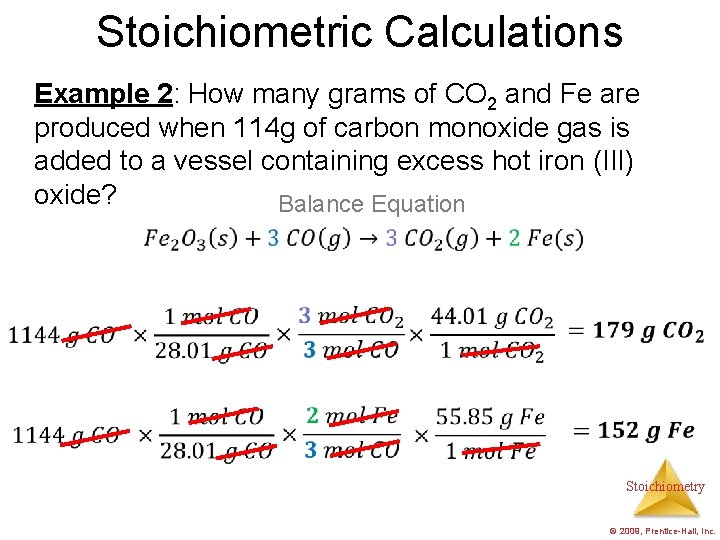Stoichiometric Calculations Example 2: How many grams of CO 2 and Fe are produced when 114 g of carbon monoxide gas is added to a vessel containing excess hot iron (III) oxide? Balance Equation Stoichiometry © 2009, Prentice-Hall, Inc.Limiting Reactants Stoichiometry © 2009, Prentice-Hall, Inc.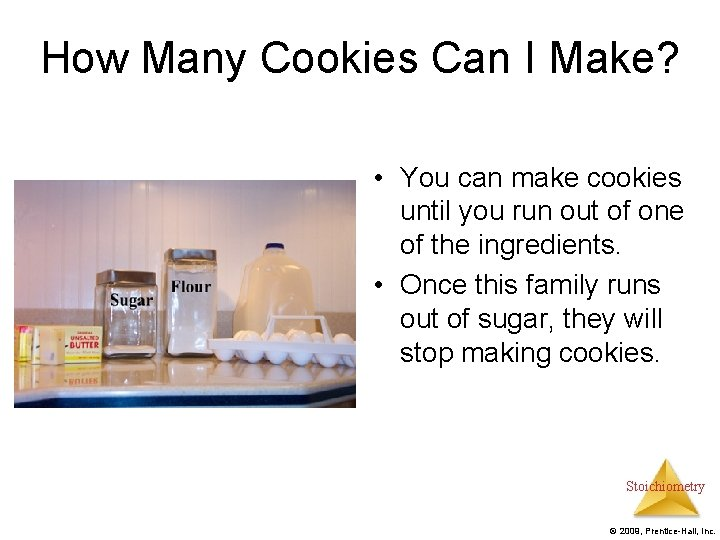How Many Cookies Can I Make? • You can make cookies until you run out of one of the ingredients. • Once this family runs out of sugar, they will stop making cookies. Stoichiometry © 2009, Prentice-Hall, Inc.How Many Cookies Can I Make? • In this example the sugar would be the limiting reactant, because it will limit the amount of cookies you can make. Stoichiometry © 2009, Prentice-Hall, Inc.Limiting Reactants • The limiting reactant is the reactant present in the smallest stoichiometric amount. – In other words, it’s the reactant you’ll run out of first (in this case, the H 2). Stoichiometry © 2009, Prentice-Hall, Inc.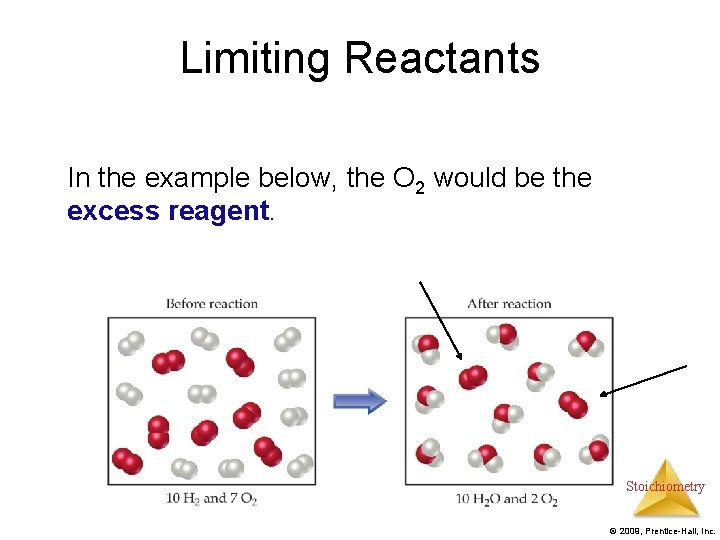Limiting Reactants In the example below, the O 2 would be the excess reagent. Stoichiometry © 2009, Prentice-Hall, Inc.Limiting Reagent Example 1: (a) What is the limiting reactant when 28 g of glucose reacts with 14 g of oxygen gas? (b) What mass of CO 2 is produced? Step 1: Balance Equation Stoichiometry © 2009, Prentice-Hall, Inc.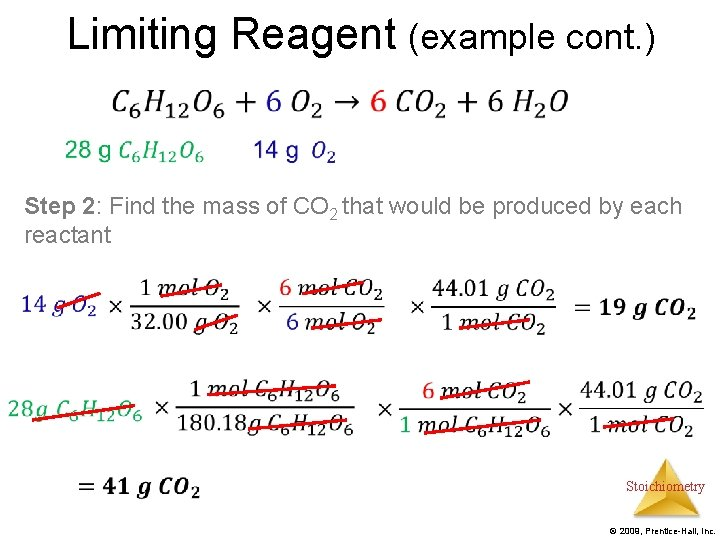Limiting Reagent (example cont. ) • Step 2: Find the mass of CO 2 that would be produced by each reactant Stoichiometry © 2009, Prentice-Hall, Inc.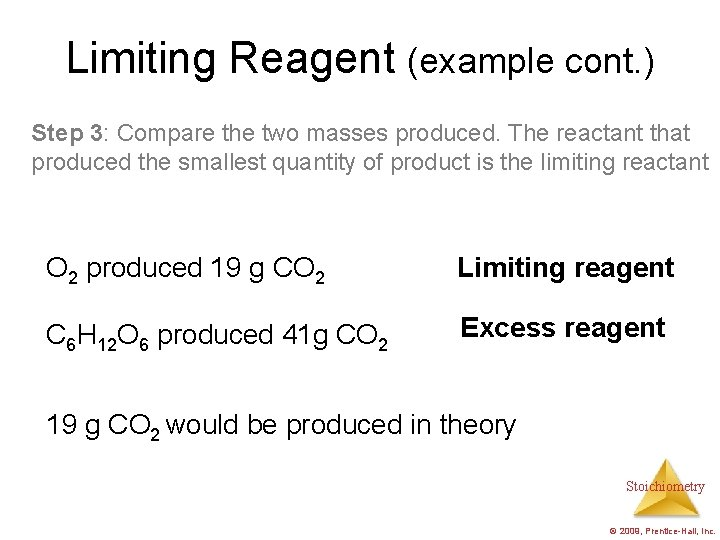Limiting Reagent (example cont. ) Step 3: Compare the two masses produced. The reactant that produced the smallest quantity of product is the limiting reactant O 2 produced 19 g CO 2 Limiting reagent C 6 H 12 O 6 produced 41 g CO 2 Excess reagent 19 g CO 2 would be produced in theory Stoichiometry © 2009, Prentice-Hall, Inc.Limiting Reagent Example How many grams of water will be produced if 150 g of hydrogen gas and 1500 grams of oxygen gas are used in the following unbalanced reaction H 2(g) + O 2(g) H 2 O Stoichiometry © 2009, Prentice-Hall, Inc.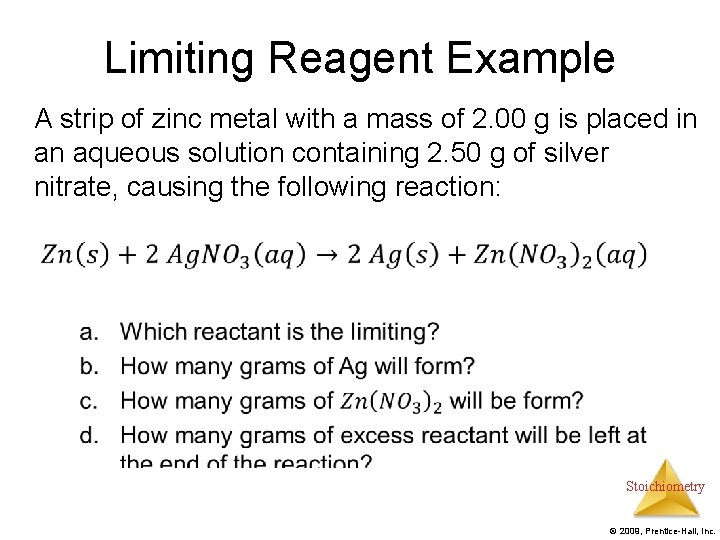Limiting Reagent Example A strip of zinc metal with a mass of 2. 00 g is placed in an aqueous solution containing 2. 50 g of silver nitrate, causing the following reaction: Stoichiometry © 2009, Prentice-Hall, Inc.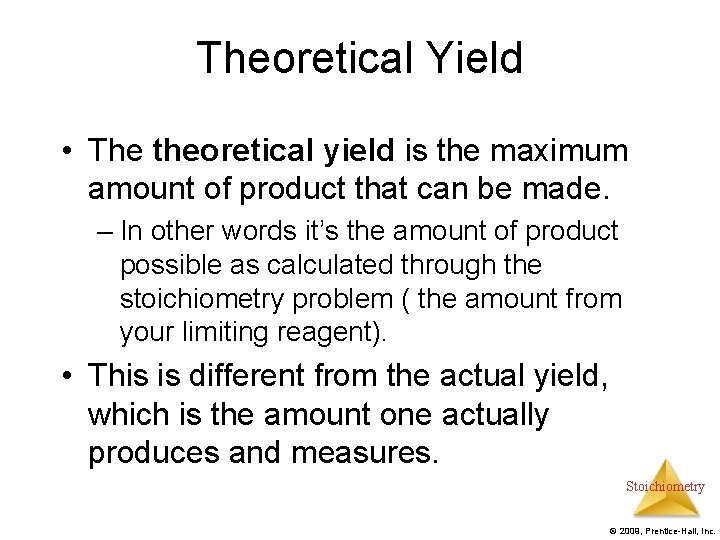Theoretical Yield • The theoretical yield is the maximum amount of product that can be made. – In other words it’s the amount of product possible as calculated through the stoichiometry problem ( the amount from your limiting reagent). • This is different from the actual yield, which is the amount one actually produces and measures. Stoichiometry © 2009, Prentice-Hall, Inc.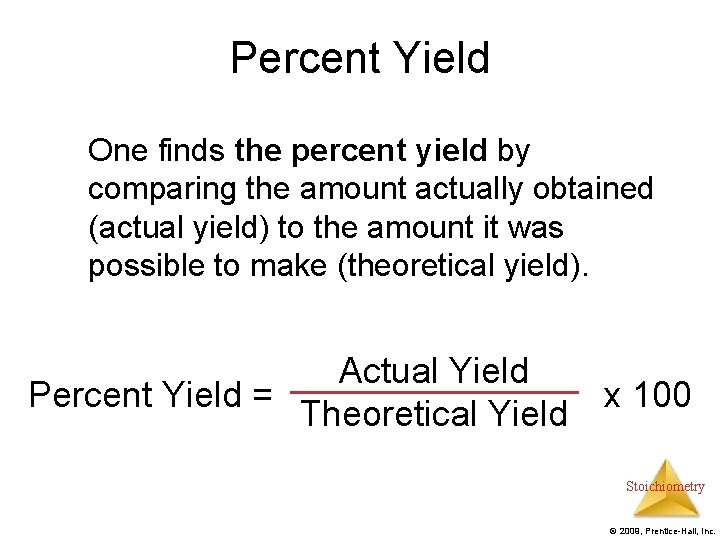Percent Yield One finds the percent yield by comparing the amount actually obtained (actual yield) to the amount it was possible to make (theoretical yield). Actual Yield Percent Yield = x 100 Theoretical Yield Stoichiometry © 2009, Prentice-Hall, Inc.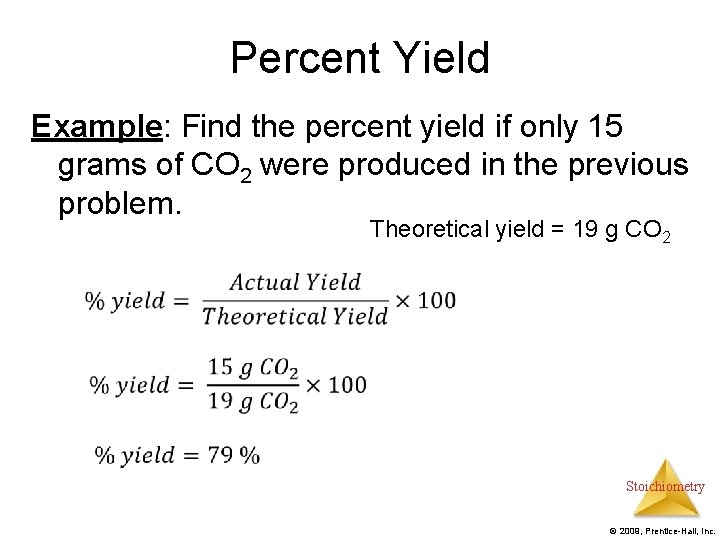Percent Yield Example: Find the percent yield if only 15 grams of CO 2 were produced in the previous problem. Theoretical yield = 19 g CO 2 Stoichiometry © 2009, Prentice-Hall, Inc.